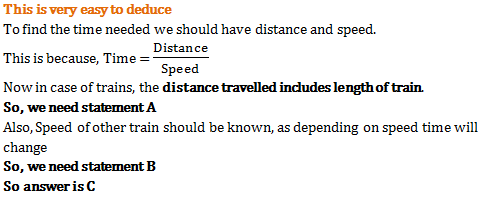# Problems on Trains - Quantitative Aptitude (MCQ) questions for Q. 29268

Q.  Consider statements A and B given below and decide whether they are enough to find the time needed for two trains to cross each other when they are travelling in opposite directions and one train is moving at a speed of 86 km/hr.

Statement A – Trains are of equal length of 350m
Statement B – Other train is moving with speed 83 km/hr

- Published on 11 Apr 17

a. Only statement B is enough
b. Any one statement if given is enough to find the answer
c. Both statements together are needed to find the answer
d. Even both statements together are not enough to find the answer